# Looking Up Information in a Data List

Whenever you create a worksheet that holds information about a list of distinct items, such as products offered for sale by a company, you should ensure that at least one column in the list contains a unique value that distinguishes that row (and the item the row represents) from every other row in the list. Assigning each row a column with a unique value means that you can associate data in one worksheet with data in another worksheet. For example, if every customer is assigned a unique identification number, you can store a customer's contact information in one worksheet and all orders for that customer in another worksheet. You can then associate the customer's orders and contact information without writing the contact information in a worksheet every time the customer places an order.

In the case of shipments handled by Consolidated Messenger, the column that contains those unique values, or the primary key column, is the ShipmentID column.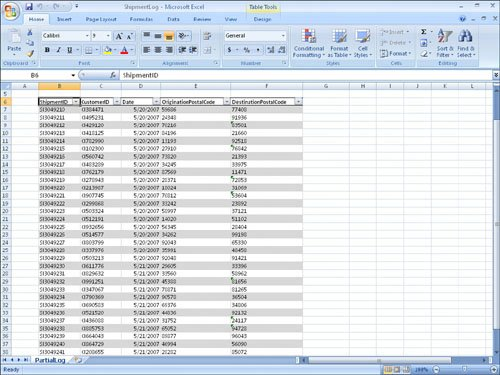If you know a shipment's ShipmentID, it's no trouble to look through a list of 20 or 30 items to find a particular shipment. If, however, you have a list of many thousands of shipments, looking through the list to find one would take quite a bit of time. Instead, you can use the VLOOKUP function to let your colleagues type a ShipmentID in a cell and have the corresponding details appear in another cell.

The VLOOKUP function finds a value in the leftmost column of a named range, such as a table, and then returns the value from the specified cell to the right of the cell with the found value. A properly formed VLOOKUP function has four arguments (data that is passed to the function), as shown in the following definition: =VLOOKUP(lookup_value, table_array, col_index_num, range_lookup).

The following table summarizes the values Excel 2007 expects for each of these arguments.

Argument

Expected Value

lookup_value

The value to be found in the first column of the named range specified by the table_array argument. The lookup_value argument can be a value, a cell reference, or a text string.

table_array

The multicolumn range or name of the range or data table to be searched.

col_index_num

The number of the column in the named range with the value to be returned.

range_lookup

A TRUE or FALSE value, indicating whether the function should find an approximate match (TRUE) or an exact match (FALSE) for the lookup_value. If left blank, the default value for this argument is TRUE.

Important

When range_lookup is left blank or set to TRUE, for VLOOKUP to work properly the rows in the named range specified in the table_array argument must be sorted in ascending order based on the values in the leftmost column of the named range.

The VLOOKUP function works a bit differently depending on whether the range_lookup argument is set to TRUE or FALSE. The following list summarizes how the function works based on the value of range_lookup:

• If the range_lookup argument is left blank or set to TRUE, and VLOOKUP doesn't find an exact match for lookup_value, the function returns the largest value that is less than lookup_value.

• If the range_lookup argument is left blank or set to TRUE, and lookup_value is smaller than the smallest value in the named range, an #N/A error is returned.

• If the range_lookup argument is left blank or set to TRUE, and lookup_value is larger than all values in the named range, the largest value in the named range is returned.

• If the range_lookup argument is set to FALSE, and VLOOKUP doesn't find an exact match for lookup_value, the function returns an #N/A error.

As an example of a VLOOKUP function, consider the following worksheet and the accompanying VLOOKUP formula.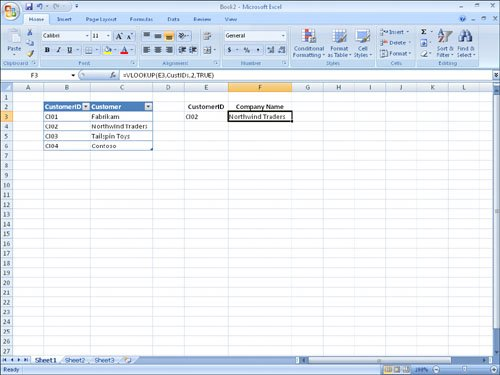Tip

The related HLOOKUP function matches a value in a column of the first row of a table and returns the value in the specified row number of the same column. For more information on using the HLOOKUP function, click the Excel 2007 Help button, type HLOOKUP in the search terms box, and then click Search.

When you type CI02 in cell E3 and press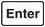, the VLOOKUP function searches the first column of the table, finds an exact match, and returns the value Northwind Traders to cell C3.

Caution

Be sure to give the cell in which you type the VLOOKUP formula the same format as the data you want the formula to display. For example, if you create a VLOOKUP formula in cell G14 that finds a date, you must apply a date cell format to cell G14 for the formula's result to display properly.

In this exercise, you create a VLOOKUP function to return the destination postal code of deliveries with ShipmentIDs typed in a specific cell.

USE the ShipmentLog workbook in the practice file folder for this topic. This practice file is located in the My Documents\Microsoft Press\Excel SBS\Database folder.

BE SURE TO start Excel 2007 before beginning this exercise.

OPEN the ShipmentLog workbook.

 1 In cell C3, type the formula =VLOOKUP(B3,Shipments,5,FALSE). Cell B3, which the formula uses to look up values in the Shipments table, is blank, so the #N/A error code appears in cell C3. 2 In cell B3, type SI3049224, and press. The value 51102 appears in cell C3. 3 In cell C3, edit the formula so that it reads =VLOOKUP(B3,Shipments,2,FALSE). The formula now finds its target value in table column 2 (the CustomerID column), so the value CI512191 appears in cell C3. 4 In cell C3, edit the formula so that it reads =VLOOKUP(B3,Shipments,4,TRUE). Changing the last argument to TRUE enables the VLOOKUP formula to find an approximate match for the ShipmentID in cell B3, whereas changing the column to 4 means the formula gets its result from the OriginationPostalCode column. The value 14020 appears in cell C3.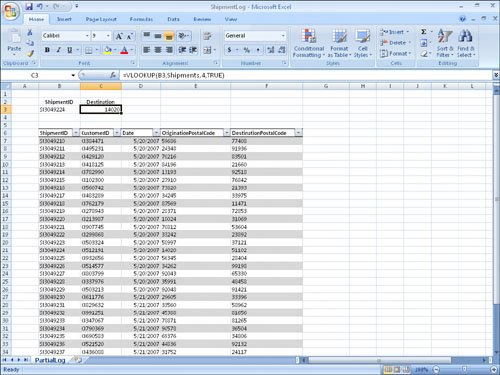5 In cell B3, type SI3049209. The value in cell B3 is smaller than the smallest value in the Shipments table's first column, so the VLOOKUP formula displays the #N/A error code in cell C3. 6 In cell B3, type SI3049245. The ShipmentID typed into cell B3 is greater than the last value in the table's first column, so the VLOOKUP formula displays the last value in the target column (in this case, the fourth column). Therefore, the value 44493 appears in cell C3.

CLOSE the ShipmentLog workbook.

CLOSE Excel 2007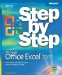MicrosoftВ® Office ExcelВ® 2007 Step by Step (Step By Step (Microsoft))
ISBN: 073562304X
EAN: 2147483647
Year: 2004
Pages: 143
Authors: Curtis Frye

Similar book on Amazon

flylib.com © 2008-2017.
If you may any questions please contact us: flylib@qtcs.net